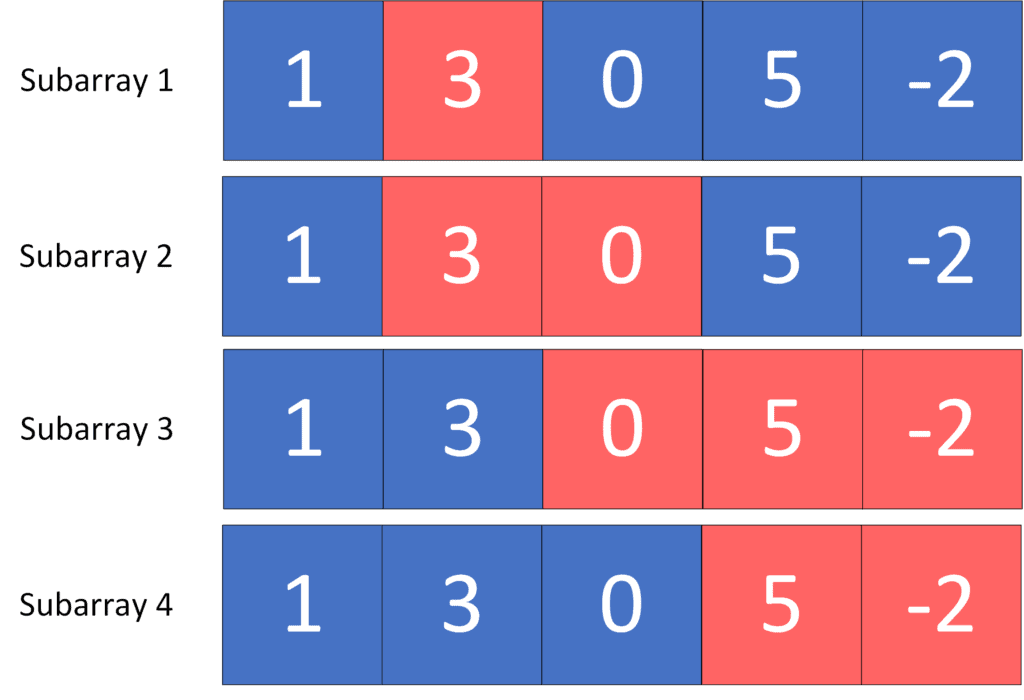## 1. Overview

In this tutorial, we’ll talk about the problem of finding the number of subarrays with a given sum.

First, we’ll define the problem and provide an example to explain it. Then, we’ll present two different approaches to solving it and work through their implementations and time complexity.

## 2. Defining the Problem

Suppose we have an array, and we were asked to count the number of subarrays with a sum equal to.

Recall that a subarray of an array is a consecutive range of the array elements.

Let’s take a look at the following example:

Given an arrayand a desired sum(red cells define a subarray with sum equal to):As we can see, the answer here is four because there aresubarrays with a sum equal to.

## 3. Naive Approach

### 3.1. Main Idea

The main idea in this approach is to check for each possible subarray if its sum is equal to, or not.

Initially, we’ll go through every possible start of a subarray. Then, we’ll fix it for each start and try all the possible ends. Next, while we’re looking for a valid end, we’ll add the current number to the sum of that subarray. If the sum of the current subarray is equal toat some moment, we’ll increase the answer by one. Otherwise, we’ll ignore that subarray.

Finally, the answer will be equal to the number of subarrays with a sum equal to.

### 3.2. Algorithm

Let’s take a look at the implementation of the algorithm:

``

Initially, we declare thefunction that will return the number of subarrays with a sum equal to. The function will have two parameters,, which represents the given array, and, the desired sum.

Initially, we declare the, which will store the number of valid subarrays. Next, we’ll try to start a new subarray from each position of the given array. Also, we declare, which will store the sum of the subarray that will begin at.

Then, we’ll iterate over all the possible subarray ends. While we move the end pointer, we’ll add the element at the end to theof the current subarray. After that, we’ll check if the current subarray is valid by checking if theis equal to. If so, we increase theby one. Otherwise, the current subarray is not valid.

Finally, thewill equal the number of subarrays that have a sum equal to.

### 3.3. Complexity

The time complexity of this algorithm is, whereis the length of the given array. This complexity is because we fixed it as a start of a subarray for each position of the given array and iterated overall positions that could be an end to that subarray.

## 4. Prefix Sum Approach

### 4.1. Main Idea

The main idea in this approach is to get the advantage of the prefix sum technique (Recallstores the sum of all elements from the beginning of the array until index).

Initially, we’ll calculate the prefix sum of the given array. Next, for each given array position, we’ll try to fix it as an end to the subarray and get the count of possible subarray starts that make the subarray valid. We can represent the valid starts that give us a valid subarrays end at the fixed position in the following formula:Therefore, the number of valid subarray starts that could end at the current position will equal the count of the prefix sum values of positions that come before the. Then, while we’re moving thepointer, we’ll increase theof the prefix sum value at that position.

Finally, thewill represent the number of subarrays with a sum equal to.

### 4.2. Algorithm

Let’s take a look at the implementation of the algorithm:

``

Initially, we have the samefunction as the previous approach.

First, we declare thearray, which will store the sum of all elements from the beginning of the given array up to a specific position. We compute theof the given arrayby applying the formula.

Second, we declare, which will store the number of valid subarrays. Furthermore, we declare thehashmap, which will store the count of occurrences of a specificvalue.

Third, we iterate over each position and fix it as the end of the subarray, and we will want to get the count of all possible subarray starts. The subarray that ends at positionwill have a valid start, if, and only if,. So, we’ll add to thethe number of positions with aequal to. After that, we’ll increase theof theby one.

Finally, thewill equal the number of subarrays that have a sum equal to.

### 4.3. Complexity

The time complexity of this algorithm is, whereis the length of the given array. This complexity is because we iterate over each element only once.

Note: the previous time complexity could be multiplied by a constant factor representing the hashmap operations’ complexity.

## 5. Conclusion

In this article, we discussed the problem of finding the number of subarrays with a given sum. We provided an example to explain the problem and explored two different approaches to solve it, where the second one had a better time complexity.

Finally, we walked through their implementations and explained the idea behind each of them.